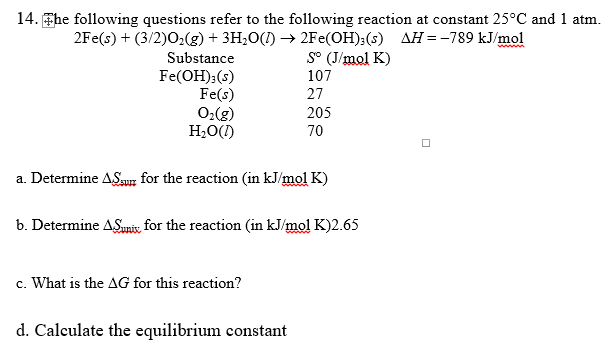# 14.he following questions refer to the following reaction at constant 25°C and 1 atm. 2Fe(s) (3/2)Og(g) + 3H20() → 2Fe(OH)3(s) A =-789 kJ/mol Substance Fe(OH)3(s) Fe(s) O2(g) H20D) So (J/mol K) 107 27 205 70 a. Determine ASsu for the reaction (in kJ/mol K) b. Determine Δ&ns, for the reaction (in kJ/mol K)2.65 c. What is the ΔG for this reaction? d. Calculate the equilibrium constant

Questionhelp_outlineImage Transcriptionclose14.he following questions refer to the following reaction at constant 25°C and 1 atm. 2Fe(s) (3/2)Og(g) + 3H20() → 2Fe(OH)3(s) A =-789 kJ/mol Substance Fe(OH)3(s) Fe(s) O2(g) H20D) So (J/mol K) 107 27 205 70 a. Determine ASsu for the reaction (in kJ/mol K) b. Determine Δ&ns, for the reaction (in kJ/mol K)2.65 c. What is the ΔG for this reaction? d. Calculate the equilibrium constant fullscreen

### Want to see this answer and more?

Experts are waiting 24/7 to provide step-by-step solutions in as fast as 30 minutes!*

*Response times vary by subject and question complexity. Median response time is 34 minutes and may be longer for new subjects.
Tagged in
ScienceChemistry

### Chemical Thermodynamics